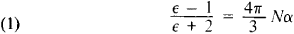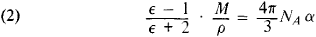# Clausius-Mossotti Equation

## Clausius-Mossotti Equation

an equation that expresses the dependence of the static dielectric constant ∊ of a nonpolar dielectric on the polarizability α of its molecules, atoms, or ions and on their number N in 1 cm3 (if the dielectric consists of particles of one type):The Clausius-Mossotti equation is frequently written in the formwhere M is the molecular weight of the substance, ρ is its density, and NA is Avogadro’s number. The right-hand side of equation (2) is sometimes called molar polarization. The equation was formulated in 1879 by the German physicist R. Clausius, who developed the ideas of the Italian scientist O. F. Mossotti.

The Clausius-Mossotti equation is strictly realized for nonpolar gases at low pressures (less than 200–500 mm Hg, or 26.6–66.5 kilonewtons per sq m [kN/m2]) or medium pressures (from 500 mm Hg to 5 atm, or 66.5–500 kN/m2). The equation is approximately realized for nonpolar gases at high pressures (above 5–10 atm, or 0.5–1 MN/m2), for nonpolar liquids, and for many nonpolar crystals.

For visible light (high-frequency electric field), the dielectric constant is equal to the square of the refractive index: ∊ = n2. The relationship between the electronic polarizability and ∊ in such fields is described by the Lorentz-Lorenz law.

References in periodicals archive ?
Table 2 compares the theoretical results of the Clausius-Mossotti equation with the values obtained using the proposed unit cell approach.
Permittivity values computed using the unit cell approach and the Clausius-Mossotti equation at 30 GHz.
The Clausius-Mossotti equation was developed for noninteracting, nonpolar molecules governed by the Lorentz equation for the internal field.
If we consider the above Clausius-Mossotti equation

Site: Follow: Share:
Open / Close